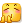# CompletableFuture

Java 8 的 CompletableFuture

### 创建

• 创建一个已完成的
• 类似 guava 的 SettableFuture, 先创建一个空的，再在合适的地方完成它
• 异步计算或运行
```private static void create() {
// 新建一个已经完成的
CompletableFuture.completedFuture("");
// JDK 9+
// CompletableFuture.failedFuture(new Exception());

// 类似 SettableFuture.create() 新建一个壳子
CompletableFuture<String> hello = new CompletableFuture<>();
// 然后在合适的时机使它完成
hello.complete("");
hello.completeExceptionally(new Exception());

// 异步运行
CompletableFuture.runAsync(() -> System.out.println("CompletableFuture<Void>"));
// 异步计算
CompletableFuture.supplyAsync(() -> "CompletableFuture<String>");
}```

### 常用操作

• thenApply 完成后对类型 T 的结果执行转换为类型 U 的操作，返回新的 Future<U>
• thenAccept 完成后对类型 T 的结果执行消费操作，返回新的 Future<Void>
• thenAcceptBoth 本类型 T 和另一个类型 U 都完成后，执行消费操作，返回 Future<Void>
• thenCombine 本类型 T 联合另一个 U 都完成后，执行一个 BiFunction<T,U,V>，返回新的 Future<V>
• thenCompose 完成后的结果 T 转换为另一个 Future<U>, 类似 flatMap
• exceptionally 异常时返回兜底结果
• whenComplete 当完成或异常时的处理，返回 Future<Void>
• handle 类似 thenApply, 返回新的 Future<U>
• 各种 Async 后缀的版本：异步执行，传入线程池。不传 Executor 则默认为 ForkJoinPool.commonPool
```private static void then() {
CompletableFuture.completedFuture("")
// 完成后执行一个 Function 进行数据转换 类似 Stream.map
.thenApply(String::length)
// 联合另一个 CompletableFuture，都完成后执行一个 BiFunction 进行数据转换
.thenCombine(CompletableFuture.completedFuture(1), (length, num) -> "len+num=" + length + num)
// 当两个都完成后执行一个动作 返回新的 CompletableFuture<Void>
.thenAcceptBoth(CompletableFuture.completedFuture(2), (s, num) -> System.out.printf("%s,%d\n", s, num))
.thenApply(v -> "s")
// 将完成后的结果转换为另一个 CompletableFuture 类似 Stream.flatMap
.thenCompose(s -> CompletableFuture.completedFuture("compose:" + s))
// 当出现异常时 处理异常并返回异常时的值
.exceptionally(e -> "之前是什么类型这里就需要返回什么类型")
// 完成后执行的动作 返回新的 CompletableFuture<Void>
.thenAccept(System.out::println)
// 类似 Accept 完成后执行的动作，返回新的 CompletableFuture<Void>
.whenComplete((result, ex) -> {
})
// 类似 Apply 完成后执行的动作，返回新的 CompletableFuture<U>
.handle((result, ex) -> "new")
;
}```

### 后续的操作在哪个线程执行？

```private static void thread() {
CompletableFuture<String> f = new CompletableFuture<>();
CompletableFuture<Long> other = new CompletableFuture<>();
f
.thenApply(s -> {
// 这里的线程是给 f 设置结果的线程
return s;
})
.thenApplyAsync(s -> {
// Async 结尾的方法不传 线程池则是 ForkJoinPoll.commonPoll
return s;
})
.thenAccept(s -> {
// 和上个操作是同一个线程
})
.thenCompose(v -> other)
.thenApply(num -> {
// 执行线程 取决于哪个 future 后完成
return num + 1;
})
;

// sleep(50);
f.complete("Ha");

sleep(50);
other.complete(1L);
}

private static void sleep(long mills) {
try {
} catch (InterruptedException e) {
e.printStackTrace();
}
}```

### 内部原理

```private static void debug() {
// 实际开发一般都是一条链走到底，这里为了 Debug 好对比哪个实例是哪个 故分开写变量
CompletableFuture<String> hello = new CompletableFuture<>();
CompletableFuture<Void> print = hello.thenAccept(System.out::println);
CompletableFuture<String> upper = hello.thenApply(String::toUpperCase);
CompletableFuture<Void> v1 = upper.thenAccept(System.out::println);
CompletableFuture<Void> v2 = print.thenCombine(upper, (aVoid, s) -> s.toCharArray())
.thenCompose(chars -> CompletableFuture.completedFuture(chars.length))
.thenAccept(System.out::println);

hello.complete("Hello");
}```发现一篇源码解析：https://www.cnblogs.com/aniao/p/aniao_cf.html